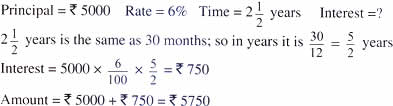Tuesday , July 5 2022# NCERT 5th Class (CBSE) Mathematics: Application Of Percentage

(b) Principal = Rs. 3000, Rate = 6%, Time = 9 months, Interest = ?

P × R × T/100 = interest

The time units must be the same when using the formula. The rate is given per year, but the time is given in months. So, we must divide the number of months by 12 to get the time in years.

3000 × 6/100 × 9/12 = 135

Interest = Rs. 135

(c) Principal = Rs. 15,000, Rate = 9%, Time = 73 days, Interest = ?

P × R × T/100 = Interest

The time units must be the same while calculating interest. The rate is given per year, but the time is given in days. So we must divide the number of days by 365 to get the time in years.

15000 × 9/100 × 73/365 = 270

So, interest = Rs. = 270

(d)Interest = 5000 × 6/100 × 5/2 = Rs. 750

Amount = Rs. 5000 + Rs. 750 = 5750

(e) Rate of interest = 6%, Time = 2 years, Interest = Rs. 3600, Principal = ?

Principal = Interest ×100/Rate × Time

Principal = 3600 × 100/6 × 2 = Rs. 30,000

## चुनौती हिमालय की 5th NCERT CBSE Hindi Rimjhim Ch 18

चुनौती हिमालय की 5th Class NCERT CBSE Hindi Book Rimjhim Chapter 18 प्रश्न: लद्दाख जम्मू-कश्मीर राज्य में …